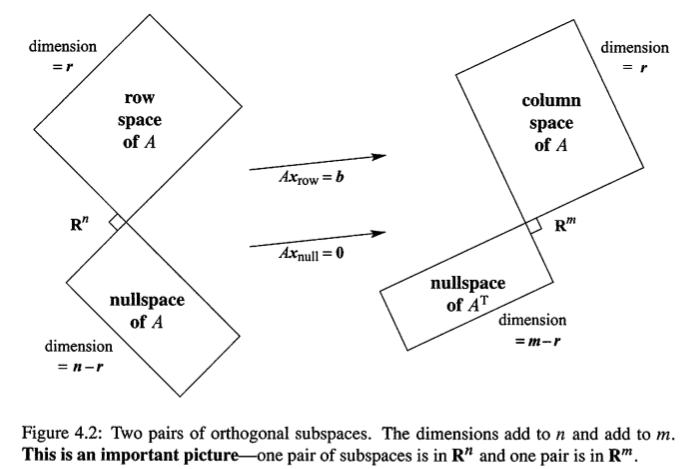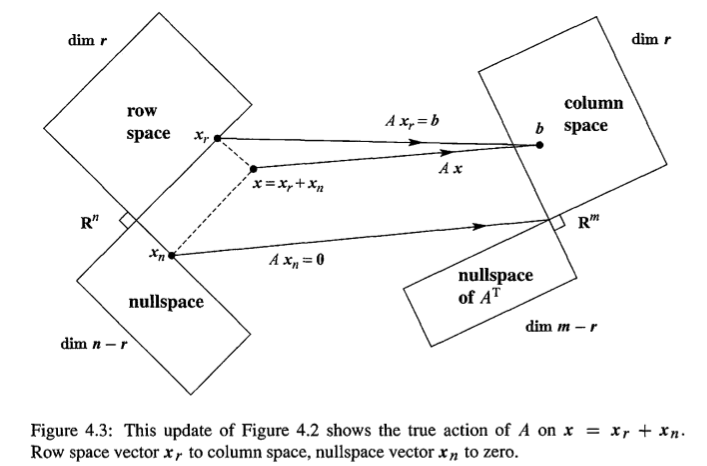Abstract: 本篇介绍正交性，向量正交，矩阵正交，子空间正交
Keywords: Orthogonality,Four Subspace,Orthogonal Complements,Fundamental Theorem of Linear Algebra ,Combining Bases from Subspaces,Split

# 四个正交子空间

## 正交

1. This is only a number
2. It is combination of column vectors
3. It shows Subspaces

1. 向量正交
2. 矩阵正交
3. 子空间正交

$$v^tw=0 \,\, and \,\, ||v||^2+||w||^2=||v+w||^2$$

definition:
$$v^tw=0 \,\,for\,\,all\,\,v\,\,in\,\,V\,\,and\,\,all\,\,w\,\,in\,\,W$$

$$r^tAx=r^t(Ax)= r^t\begin{bmatrix} \dots a_1\dots\\ \dots a_2\dots \\ \vdots\\ \dots a_n\dots \end{bmatrix} \begin{bmatrix} x_1\\ x_2\\ \vdots\\ x_n \end{bmatrix}= r^t\begin{bmatrix} 0\\ 0\\ \vdots\\ 0 \end{bmatrix}$$

$$r^tAx=0$$

Fundamental Theorem Part II（不完整版）
The row space is perpendicular to the nullspace
The column space is perpendicular to the nullspace of $A^T$

Fundamental Theorem I 说的是四个subspaces之间的维度的相互关系，第二部分说的是四个subspaces之间的正交关系，看来线性代数的核心是这四个subspace没错了。

## 正交互补(Orthogonal Complements)

This is very important ,The Fundamental subspace are more than just orthogonal in pairs.Their dimansions are also right.说实话，right这个词我想了半天也不知道对应中文那个词，三维空间中的两条线正交，但是他们并不可能是一个属于$3\times 3$矩阵的nullspace和rowspace因为他们都是dimension 1的加起来并不是3？你要问我为什么，往下看喽

Definition:Orthogonal Complement of a subspace V contains every vector that is perpendicular to V.This orthogonal subsapce is denot $V^{\perp}$(pronounced “V perp”)Fundamental Theorem Part II（完整版）
$N(A)$ is the orthogonal complement of the row space $C(A^T)$ (in $R^n$)
$N(A^T)$ is the orthogonal complement of the row space $C(A)$ (in $R^m$)

$$x=x_{particular}+x_n$$0%